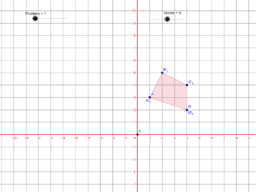# Transformation (Metamorphosis)

Author:
Mr. Watson
If you are a student this is: A magic training unit. You will learn how to perform spells such as verto figura and many others. Notice the arrows on the top of the page? Use these arrows to move between pages. You could also use the verto cera spell instead if you already know how to use it. Click on the right arrow at the top, or click on "Exploring Translations 1" to get started. Good luck! If you are a teacher this is: A less formal exploration of rigid transformations intended as a nearly self-contained 2 hour long lesson for use in an introduction to high school geometry unit. This lesson addresses (in an introductory rather than comprehensive fashion) the following CCSM standards: CCSS.MATH.CONTENT.HSG.CO.A.1 Know precise definitions of angle, circle, perpendicular line, parallel line, and line segment, based on the undefined notions of point, line, distance along a line, and distance around a circular arc. CCSS.MATH.CONTENT.HSG.CO.A.2 Represent transformations in the plane using, e.g., transparencies and geometry software; describe transformations as functions that take points in the plane as inputs and give other points as outputs. Compare transformations that preserve distance and angle to those that do not (e.g., translation versus horizontal stretch). CCSS.MATH.CONTENT.HSG.CO.A.4 Develop definitions of rotations, reflections, and translations in terms of angles, circles, perpendicular lines, parallel lines, and line segments. CCSS.MATH.CONTENT.HSG.CO.A.5 Given a geometric figure and a rotation, reflection, or translation, draw the transformed figure using, e.g., graph paper, tracing paper, or geometry software. Specify a sequence of transformations that will carry a given figure onto another. CCSS.MATH.CONTENT.HSG.GPE.B.5 Prove the slope criteria for parallel and perpendicular lines and use them to solve geometric problems (e.g., find the equation of a line parallel or perpendicular to a given line that passes through a given point). The code for the Geogebra pages is derived from user mschreiner13's sheets.